# Group algebra of a locally compact group

(diff) ← Older revision | Latest revision (diff) | Newer revision → (diff)

A topological algebra with involution formed by certain functions on the group with multiplication in it defined as convolution. Let the Banach spacebe constructed using a left-invariant Haar measure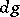on a locally compact topological groupand let the multiplication in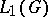be defined as the convolution; also, let the involution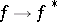be given by the formula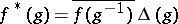, whereis the modular function of. The resulting Banach algebra with involution is said to be the group algebra ofand is also denoted by. Ifis a finite group, then the definition of the group algebra coincides with the ordinary algebraic definition of the group algebra over the field of complex numbers.

The concept of a group algebra makes it possible to use the general methods of the theory of Banach algebras in problems of group theory and, in particular, in abstract harmonic analysis. The properties of a group algebra, as a Banach algebra, reflect the properties of topological groups; thus, a group algebra contains a unit element if and only if the group is discrete; the group algebra is the direct (topological) sum of its finite-dimensional minimal two-sided ideals if and only if the group is compact. Of special importance is the concept of a group algebra in the theory of unitary representations (cf. Unitary representation) of groups: Between the continuous unitary representations of a topological groupand the non-degenerate symmetric representations (cf. Involution representation) of the group algebrathere exists a one-to-one correspondence. This correspondence puts a continuous unitary representationofin a Hilbert spaceinto correspondence with the representation ofdefined by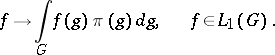Group algebras of locally compact groups have a number of properties in common. In fact, any group algebra contains an approximate unit element (cf. Banach algebra), formed by the family of characteristic functions on a neighbourhood of the unit element ordered by inclusion (in decreasing order). For this reason it is possible to establish for a group algebra a correspondence between the positive functionals on the group algebra and its symmetric representations. Any group algebra is a semi-simple algebra, and has a symmetric faithful representation. In particular, the representation of a group algebra determined by the regular representation of the group is faithful. The closed left ideals ofare the closed vector subspaces ofthat are invariant with respect to left translation.

The name group algebra is also sometimes given to the Banach algebra with involution obtained from the group algebraby the adjunction of a unit. There exist several other algebras with involutions, which are sometimes referred to as group algebras. These include, in particular: the algebra of measures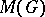with respect to convolution, algebras with respect to ordinary multiplication, such as the algebra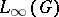of essentially-bounded functions that are measurable by the Haar measure, and the algebra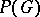spanned by the set of complex positive-definite functions. The set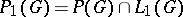and the set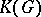of continuous functions with compact support form algebras both with respect to convolution and with respect to ordinary multiplication. One obtains the following table, in which arrows denote inclusions: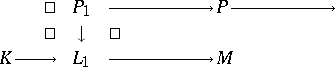How to Cite This Entry:
Group algebra of a locally compact group. Encyclopedia of Mathematics. URL: http://encyclopediaofmath.org/index.php?title=Group_algebra_of_a_locally_compact_group&oldid=16083
This article was adapted from an original article by A.I. Shtern (originator), which appeared in Encyclopedia of Mathematics - ISBN 1402006098. See original article